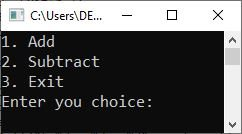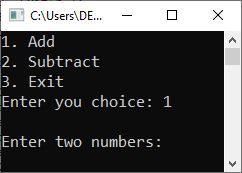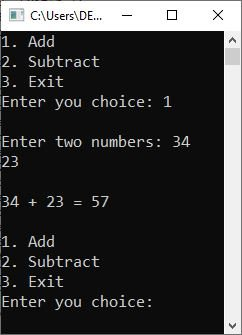# do...while loop in C programming with examples

In this post, you will learn about the C programming language's "do...while" loop. So let us begin with a brief description.

## In C, an introduction to the do...while loop

With the "do...while" loop, a block of code is run until the given condition evaluates to false. However, the block of code written inside the "do...while" loop executes once even if the condition evaluates to false at first evaluation. This is why the condition is written at the bottom of the loop.

## When should we use the do...while loop in C?

If you want to execute a block of code at once even if the given condition evaluates to false at first evaluation, then go for the "do while" loop.

## General form of the do...while loop in C

The general form of the "do...while" loop in C programming is as follows:

```do
{
// body of the "do...while" loop
} while(condition);```

After the "body of the do...while loop" executes, the "condition" is evaluated. If the condition is true, the program flow returns to the body of the "do while" loop. After executing all of the code written in the loop's body, the program flow returns to the condition-checking section and evaluates the condition. If the condition is evaluated again as true, the program flow returns to the loop's body. This process is repeated until the specified condition evaluates to false.

## while loop example in C

Consider the following program as an example of a "do...while" loop in the C programming language:

```#include <stdio.h>
int main()
{
int x = 10;
do
{
printf("The value of 'x' = %d", x);
} while(x<10);

return 0;
}```

The output produced by the above program is:

`The value of 'x' = 10`

You can see that the body of the "do...while" loop executes once even if the specified condition always evaluates to false.

Before we conclude our discussion of the "do...while" loop in C programming, let me provide another example that may help you understand the topic better.

```#include <stdio.h>
int main()
{
int op, a, b;

do
{
printf("2. Subtract\n");
printf("3. Exit\n");
printf("Enter you choice: ");
scanf("%d", &op);

switch(op)
{
case 1:
printf("\nEnter two numbers: ");
scanf("%d %d", &a, &b);
printf("\n%d + %d = %d", a, b, a+b);
break;
case 2:
printf("\nEnter two numbers: ");
scanf("%d %d", &a, &b);
printf("\n%d - %d = %d", a, b, a-b);
break;
case 3:
return 0;
default:
printf("\nWrong choice!");
}
printf("\n\n");

} while(op >= 1 && op <= 3);

return 0;
}```

The following snapshot shows the initial output produced by the above program.No, type your choice, say "1" and hit the ENTER key to see the following output:Now type the first number, say 34, and hit the ENTER key. Then type the second number, say 23, and hit the ENTER key to get the following output:You can now repeat the process to perform another addition operation or select another option to perform another operation. This process continues until the user chooses "3" to exit the program.

If the user chooses "3" as the option to exit the program, then the program will be successfully completed using the "return 0" statement. Otherwise, if the user chooses a number other than 1, 2, and 3, then the condition specified, which is:

`op >= 1 && op <= 3`

evaluates to false, and the execution of the "do...while" loop automatically stops.

C Online Test

« Previous Tutorial Next Tutorial »